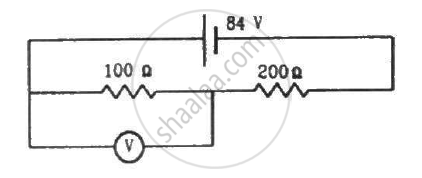Advertisement Remove all ads

# A Voltmeter of Resistance 400 ω is Used to Measure the Potential Difference Across the 100 ω Resistor in the Circuit Shown in the Figure. (A) What Will Be the Reading of the Voltmeter? - Physics

Sum

A voltmeter of resistance 400 Ω is used to measure the potential difference across the 100 Ω resistor in the circuit shown in the figure. (a) What will be the reading of the voltmeter? (b) What was the potential difference across 100 Ω before the voltmeter was connected?Advertisement Remove all ads

#### Solution

(a) The effective resistance of the circuit,

$R_{eff} = \frac{100 \times 400}{500} + 200 = 280 \Omega$

The current through the circuit,

$i = \frac{84}{280} = 0 . 3 A$

Since 100 Ω resistor and 400 Ω resistor are connected in parallel, the potential difference will be same across their ends. Let the current through 100 Ω resistor be i1 ; then, the current through 400 Ω resistor will be i - i1.

$100 i_1 = 400\left( i - i_1 \right)$

$\Rightarrow 500 i_1 = 400i$

$\Rightarrow i_i = \frac{4}{5}i = 0 . 24 A$

The reading of the voltmeter = 100 × 0.24 = 24 V

(b) Before the voltmeter is connected, the two resistors 100 Ω resistor and 200 Ω resistor are in series.

The effective resistance of the circuit,

$R_{eff} = \left( 200 + 100 \right)$

$= 300 \Omega$

The current through the circuit,

$i = \frac{84}{300} = 0 . 28 A$

∴ Voltage across the 100 Ω resistor = (0.28 × 100) = 28 V

Concept: Potential Difference and Emf of a Cell
Is there an error in this question or solution?
Advertisement Remove all ads

#### APPEARS IN

HC Verma Class 11, Class 12 Concepts of Physics Vol. 2
Chapter 10 Electric Current in Conductors
Q 48 | Page 201
Advertisement Remove all ads
Advertisement Remove all ads
Share
Notifications

View all notifications

Forgot password?